# Precalculus : Inverses of Matrices

## Example Questions

### Example Question #1 : Find The Multiplicative Inverse Of A Matrix

What is the inverse of the following nxn matrix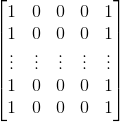The matrix is not invertible.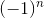The matrix is not invertible.

Explanation:

Note the first and the last columns are equal.

Therefore, when we try to find the determinant using the following formula we get the determinant equaling 0: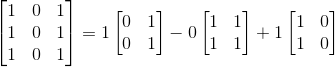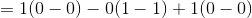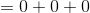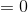This means simply, that the matrix does not have an inverse.

### Example Question #1 : Find The Multiplicative Inverse Of A Matrix

Find the inverse of the matrix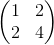.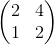Does not exist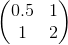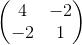Does not exist

Explanation:

For a 2x2 matrix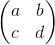the inverse can be found by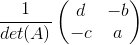Because the determinant is equal to zero in this problem, or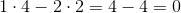,

the inverse does not exist.

### Example Question #3 : Inverses Of Matrices

Find the inverse of the matrix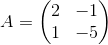.None of the other answers.Explanation:

There are a couple of ways to do this. I will use the determinant method.

First we need to find the determinant of this matrix, which is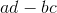for a matrix in the form:.

Substituting in our values we find the determinant to be: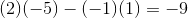Now one formula for finding the inverse of the matrix is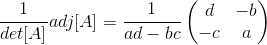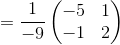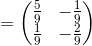.

### Example Question #4 : Inverses Of Matrices

Find the inverse of the matrix.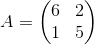Explanation:

We use the inverse of a 2x2 matrix formula to determine the answer. Given a matrix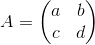it's inverse is given by the formula: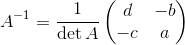First we define the determinant of our matrix: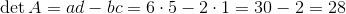Then,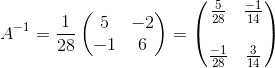### Example Question #5 : Inverses Of Matrices

Find the inverse of the following matrix.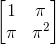This matrix has no inverse.This matrix has no inverse.

Explanation:

This matrix has no inverse because the columns are not linearly independent. This means if you row reduce to try to compute the inverse, one of the rows will have only zeros, which means there is no inverse.

### Example Question #1 : Find The Multiplicative Inverse Of A Matrix

Find the multiplicative inverse of the following matrix: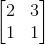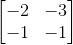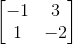This matrix has no inverse.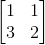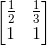Explanation:

By writing the augmented matrix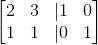, and reducing the left side to the identity matrix, we can implement the same operations onto the right side, and we arrive at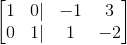, with the right side representing the inverse of the original matrix.

### Example Question #7 : Inverses Of Matrices

What is the inverse of the identiy matrix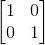?

The identity matrixThe identity matrix